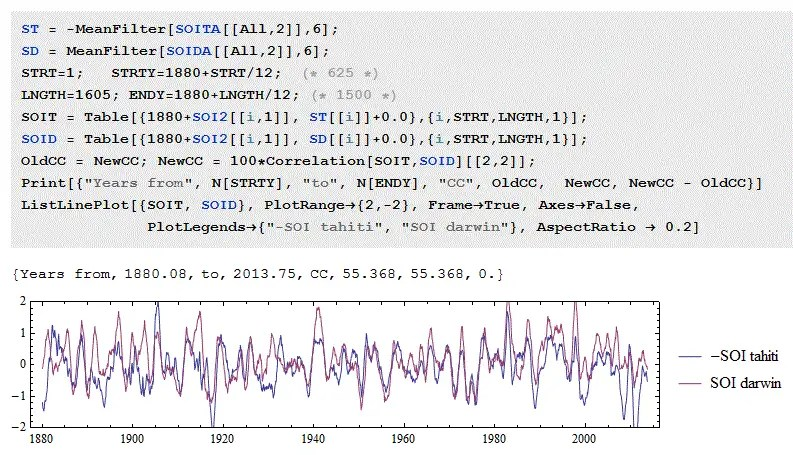# Correlation of time series

[mathjax]The Southern Oscillation embedded with the ENSO behavior is what is called a dipole , or in other vernacular, a standing wave.  Whenever the atmospheric pressure at Tahiti is high, the pressure at Darwin is low, and vice-versa.  Of course the standing wave is not perfect and far from being a classic sine wave.

To characterize the quality of the dipole, we can use a measure such as a correlation coefficient applied to the two time series.  Flipping the sign of Tahiti and applying a correlation coefficient to SOI, we get Figure 1 below:Fig 1 : Anti-correlation between Tahiti and Darwin. The sign of Tahiti is reversed to see better the correlation. The correlation coefficient is calculated to be 0.55 or 55/100.

Note that this correlation coefficient is “only” 0.55 when comparing the two time-series, yet the two sets of data are clearly aligned.  What this tells us is that other factors, such as noise in the measurements, can easily drop correlated waveforms well below unity.

This is what we have to keep in mind when evaluating correlations of data with models as we can see in the following examples.

# Sloshing Animation

The models of ENSO for SOI and proxy records apply sloshing dynamics to describe the quasi-periodic behavior. see J. B. Frandsen, “Sloshing motions in excited tanks,” Journal of Computational Physics, vol. 196, no. 1, pp. 53–87, 2004.

The following GIF animations are supplementary material from S. S. Kolukula and P. Chellapandi, “Finite Element Simulation of Dynamic Stability of plane free-surface of a liquid under vertical excitation.”

Detuning Effect.gif shows the animation of sloshing fluid for the fourth test case, with frequency ratio Ω3 = 0.5 and forcing amplitudeV = 0.2: test case 4 as shown in Figure 4. This case corresponds to instability in the second sloshing mode lying in the first instability region. Figure 8(b) shows the free-surface elevation and Figure 9 shows the moving mesh generated in this case.Dynamic Instability.gif shows the animation of sloshing fluid for the second test case which lies in the unstable region, with frequency ratio Ω1 = 0.5 and forcing amplitude kV =0.3: test case 2 as shown in Figure 4. Figure 6 shows the free-surface elevation and Figure 7 shows the moving mesh generated in this case.